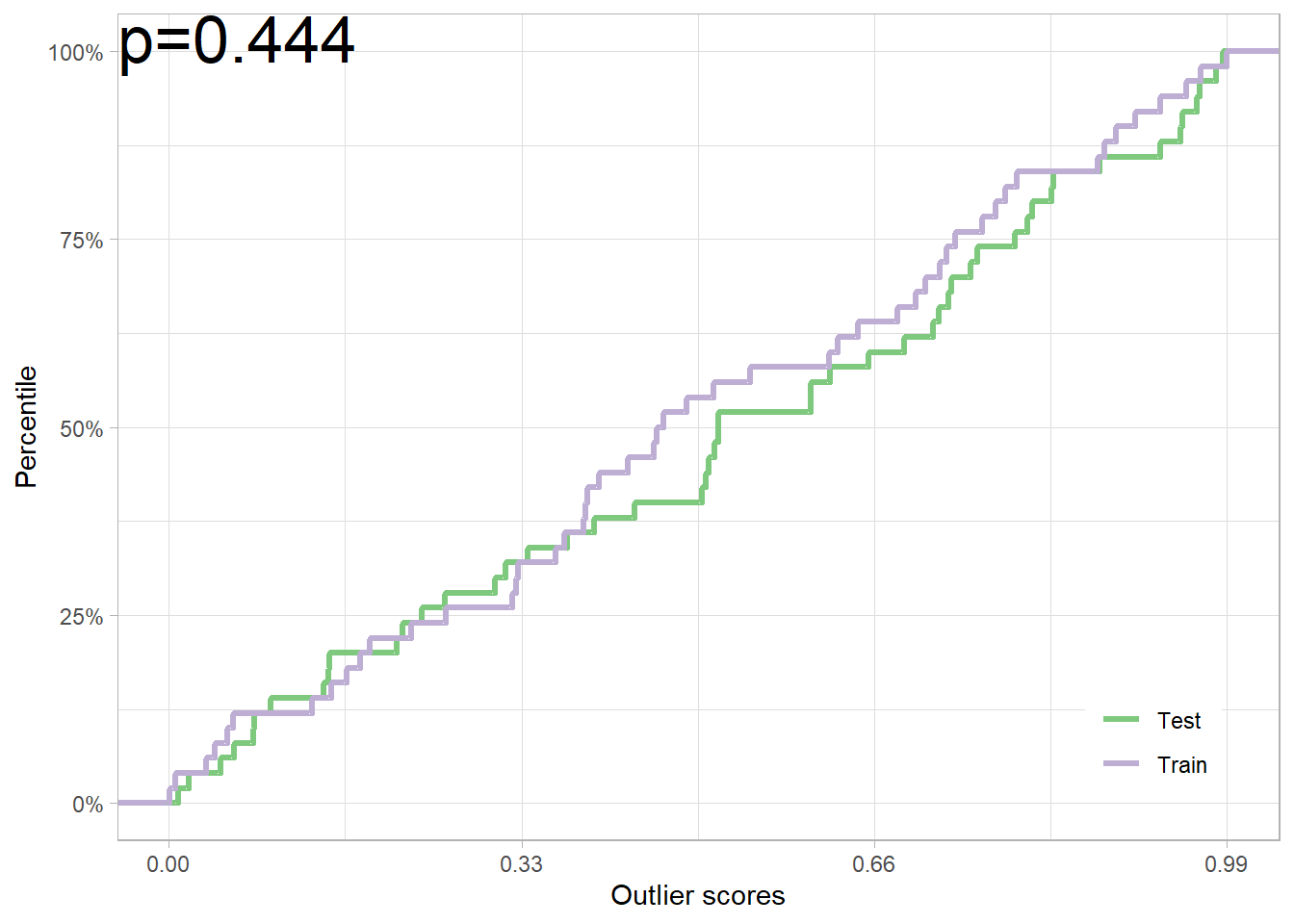Please see the paper (Kamulete 2022) for details. We denote the R package as dsos, to avoid confusion with D-SOS, the method.

## DIY

To test for adverse shift, we need two main ingredients: outlier scores from a scoring function and a way to compute a $$p-$$value (or a Bayes factor). First, the scoring function assigns to a potentially multivariate observation a univariate score. Second, for $$p-$$value, we may use permutations. The prefix pt stands for permutation test. The function pt_refit is a wrapper for this approach, provided we supply a user-defined scoring function. Sample splitting and out-of-bag variants are alternatives to permutations. Both use the asymptotic null distribution and sidestep refitting (recalibrating) the scoring function after every permutation. As a result, they can be appreciably faster than inference based on permutations.

## In Action

Take the iris dataset for example. The training set consists of different flower species. We use a completely random scoring function scorer for illustration: the outlier scores are drawn from the uniform distribution.

set.seed(12345)
data(iris)
x_train <- iris[1:50, 1:4] # Training sample: Species == 'setosa'
x_test <- iris[51:100, 1:4] # Test sample: Species == 'versicolor'
scorer <- function(tr, te) list(train = runif(nrow(tr)), test = runif(nrow(te)))
iris_test <- pt_refit(x_train, x_test, score = scorer)
plot(iris_test)dsos provides the building blocks for plugging in your own own scoring functions. The scorer function above is an example of a custom scoring function. That is, you can bring your own scores.